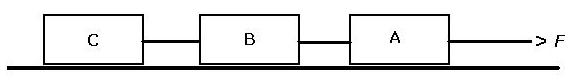AP PhysicsName _____________
John Dewey High SchoolPeriod_____________

Systems of Concurrent Straight-Line Forces
A Three-Body Problem

Solve the following problems in the spaces provided.

1. In the frictionless system illustrated in the diagram above, blocks A, B, and C possess the same mass. Here, the entire system is accelerated by an applied force
with a magnitude of F. Calculate the tension in the cord between Blocks B and C.

Base your answers to questions 2 and 3 on the diagram above and the following information: The frictionless system illustrated in the diagram above is accelerated by a force with a magnitude of F. The tension in the string connecting blocks A and B is F/2.

2. If blocks B and C each have a mass of m, determine the mass of Block A.

3. Determine the tension in the string connecting Blocks B and C.